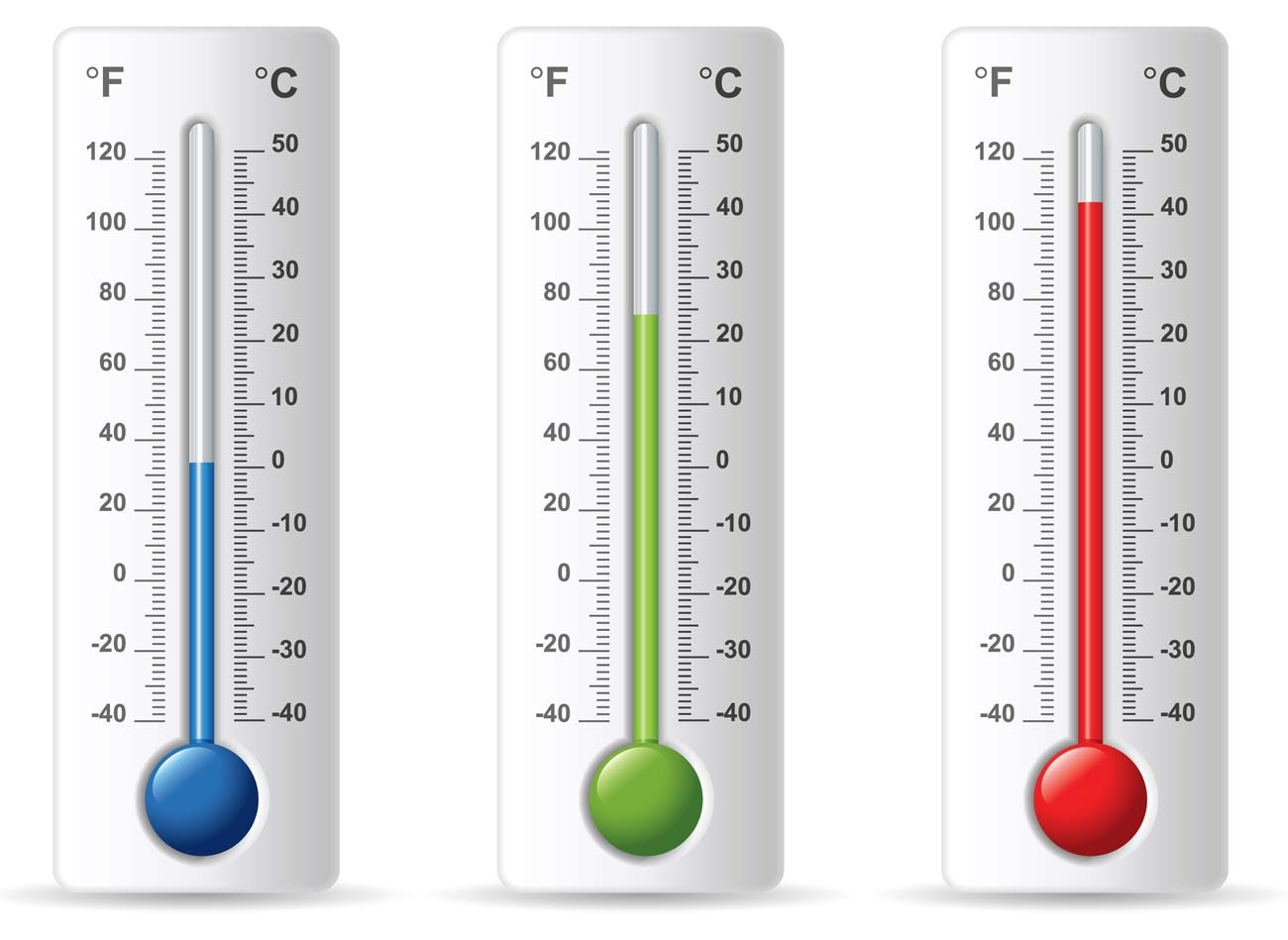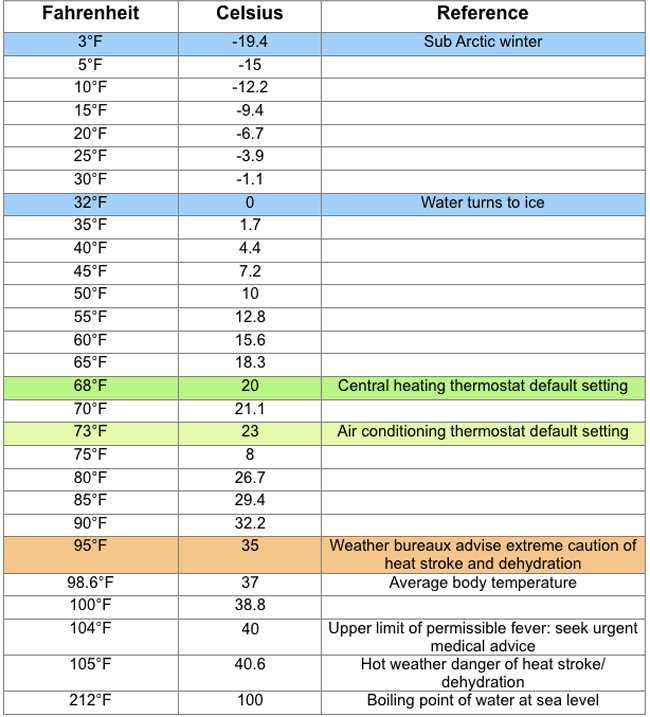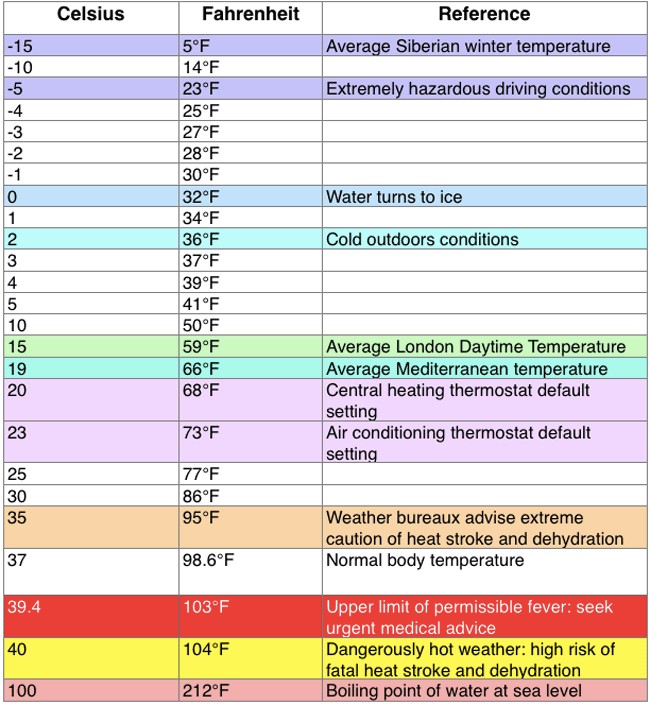# Temperature Converter: Fahrenheit to Celsius & More

Use this temperature converter to convert instantly between celsius (centigrade), fahrenheit, kelvin and other metric and imperial temperature units.

Disclaimer: Whilst every effort has been made in building our calculator tools, we are not to be held liable for any damages or monetary losses arising out of or in connection with their use. Full disclaimer.

## Converting from Fahrenheit to Celsius

From the mid-18th to mid-20th centuries, Fahrenheit was the system most widely used in English-speaking countries, while continental Europeans preferred 'The Swedish Scale'. With the drive to standardisation and metrication from the 1950s, Celsius became the adopted temperature scale for the EEC (later EU), with the UK and Ireland converting (somewhat reluctantly) after joining the European community.

This reluctance was partly due to human preference. For while the Celsius scale was developed for convenience, Fahrenheit's scale was based on observation and measurement. Also, whereas Fahrenheit had developed a ratio scale, Celsius had produced an interval scale. These are very different things, as we shall explain in a future article.

This may help explain why many people prefer Fahrenheit readings as rather closer to actual experience. In Celsius, a chilly day at 11°C does not seem greatly different from a really hot one at 35°C. On the Fahrenheit scale, these respectively measure at about 52°F and 95°F, with the latter traditionally taken as license for red top tabloids to start running 48 point headlines containing words like 'Phew', 'Flaming' and 'Scorcher'.

Incidentally, while the UK's Met Office started publishing temperatures in both Celsius and Fahrenheit from 1962 and dropping use of Fahrenheit altogether from official reports in 1970, British media persisted in using Fahrenheit in weather reports well into the 21st century. Indeed it became the fashion to use Celsius when describing extreme winter conditions and Fahrenheit for heat waves - a nice example of double standards in action.## Fahrenheit and Celsius formula

The formula to convert Fahrenheit to Celsius is:

[°C] = ([°F] - 32) × 5/9

In other words, take your Fahrenheit reading, subtract 32, multiply the answer by five and then divide by nine. So 66 degrees Fahrenheit minus 32 would be 34, times five makes 170 divided by nine equals 18.88 recurring, or 18.9°C.

## Celsius and Fahrenheit formula

[°F] = [°C] × 9/5 + 32

Here, you multiply your Celsius temperature by nine, divide by five and then add 32 to find the Fahrenheit reading.

So, 13 degrees Celsius times nine is 117, divided by five is 23.4, plus 32 makes 55.4°F.

## Using decimals

To make life a little simpler, using decimals instead of fractions, you can use 1.8 instead of 9/5.

This gives the following modified formulae

• Celsius to Fahrenheit:
(°C + 40) × 1.8 - 40 = °F
• Fahrenheit to Celsius:
(°F + 40) / 1.8 - 40 = °C

Trying this out in action on a hot day (and remembering that multiplying by 1.8 is the same as doubling and then subtracting 10 per cent) we could have 36°C plus 40 is 76, times 1.8 is 136.8 minus 40 is 96.8°F, which is firmly in 'Scorcha' territory.

And the other way, on a rather chillier day, 48°F plus 40 is 88, divided by 1.8 is 48.88 recurring (48.9) minus 40 equals 8.9°C, which actually does sound quite cold.

## Fahrenheit to Celsius reference chart

Celsius readings rounded up or down to single decimal points.## Celsius to Fahrenheit reference chart

Fahrenheit readings rounded up or down to nearest whole degreeIf you are cooking or baking, take a look at our charts for oven temperature conversions.

## Conversion units for the Temperature Converter

Celsius or Centigrade, Fahrenheit, Kelvin, Rankine, Reamur,

To help with productivity, we now set a cookie to store the last units you have converted from and to. This means that when you re-visit this temperature converter, the units will automatically be selected for you.

From abacus to iPhones, learn how calculators developed over time.

Discover the number one song and movie on the day you were born.